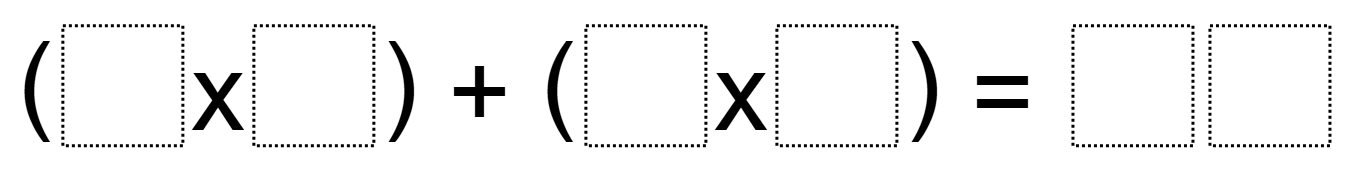Directions: Using the digits 0 to 9, at most one time each, fill in the boxes to make a true statement with the greatest possible total.### Hint

HINT GOES HERE

The greatest total we’ve found so far is 83 which comes from (9 x 7) + (5 x 4). Do you have a greater total? Let us know in the comments.

Source: Owen Kaplinsky and Robert Kaplinsky

## Product Close to 1,000

Directions: Using the digits 1 to 9 at most one time each, place a digit …

1.Traut Core Knowledge 4th Grade Ms. Petros’ class got 96. (9 x 8) + (4 x 6).

••That doesn’t work. You used the number 9 twice

2.Traut Core Knowledge 4th Grade Ms. Petros’ Class got 96. (9×8) + (6×4)

3.You are using the 9 twice. Breaking the stated rules.

4.I got 115 with ( 9 x 8 ) + ( 7 x 6 )

5.You can’t have a 3 digit product.

6.7.An exhaustive search yields the greatest answer is (9 x 7) + (5 x 4) = 83.
Here is the code:
import itertools
for trial in itertools.permutations(range(0,10),4):
max_trials = trial

and the result:

•And here are all the unique answers with a representative expression that results in the answer:
12 = (9*0) + (4*3)
14 = (9*0) + (7*2)
16 = (9*0) + (8*2)
18 = (9*2) + (7*0)
20 = (8*1) + (4*3)
21 = (9*0) + (7*3)
24 = (9*1) + (5*3)
25 = (7*3) + (4*1)
26 = (7*3) + (5*1)
27 = (9*3) + (8*0)
28 = (9*0) + (7*4)
29 = (8*3) + (5*1)
30 = (7*4) + (2*1)
32 = (9*0) + (8*4)
36 = (9*4) + (8*0)
37 = (8*4) + (5*1)
38 = (9*4) + (2*1)
39 = (8*4) + (7*1)
41 = (9*3) + (7*2)
42 = (9*0) + (7*6)
43 = (8*1) + (7*5)
45 = (7*6) + (3*1)
46 = (8*5) + (3*2)
47 = (9*5) + (2*1)
48 = (9*5) + (3*1)
50 = (9*4) + (7*2)
51 = (9*3) + (6*4)
52 = (8*6) + (4*1)
54 = (9*6) + (8*0)
56 = (9*0) + (8*7)
57 = (9*6) + (3*1)
58 = (9*6) + (4*1)
59 = (8*7) + (3*1)
60 = (9*4) + (8*3)
61 = (9*5) + (8*2)
62 = (9*3) + (7*5)
63 = (9*7) + (8*0)
65 = (9*7) + (2*1)
67 = (9*3) + (8*5)
68 = (9*7) + (5*1)
70 = (9*6) + (8*2)
72 = (9*8) + (6*0)
74 = (9*8) + (2*1)
75 = (9*8) + (3*1)
76 = (9*8) + (4*1)
81 = (9*7) + (6*3)
82 = (9*6) + (7*4)
83 = (9*7) + (5*4)

•This looks great! I’m assuming you used Python.

•My 4th grade students offer this solution:

(9 X 8) + (6 X 4) = 72 + 24 = 96

•Good try, but you used the 9 and the 6 both as factors and as digits in the product. You can’t repeat any of the digits used in the factors as digits of the product.